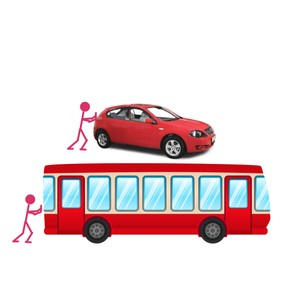Newton's Second Law of Motion

# Newton's Second Law of Motion

I can explain how an object’s mass, acceleration, and force will determine...8,000 schools use Gynzy92,000 teachers use Gynzy1,600,000 students use Gynzy

## General

Students will learn the definition of Newton’s Second Law of Motion. They will learn how an object’s mass and acceleration interact. They will also learn how the object’s acceleration is related to the force acting upon it.

NGSS: MS-PS2-2

## Learning objective

Students will be able to explain how an object’s mass, acceleration, and force will determine its movement.

## Introduction

Students are presented with a scenario where a boy is about to push his sister, who is sitting on a skateboard, down the driveway. They will think about what will happen to her speed as she is pushed. They will also imagine what would be different if the boy was pushing his father instead of his sister.

## Instruction

Students will be introduced to Newton’s Second Law of Motion and will define “mass,” “acceleration,” and “force.” They will apply these concepts to examples like a person pushing a car or a bus. They will discuss the relationship between mass and acceleration, as well as the relationship between force and acceleration. They will learn the equation for Newton’s Second Law of Motion, F = m × a, and use it to solve word problems.

## Quiz

Review what students learned about Newton’s Second Law of Motion with 5 true/false questions and 5 multiple-choice questions.

## Closing

Students will complete an exit slip with a conceptual question and a word problem.

### The online teaching platform for interactive whiteboards and displays in schools

• Save time building lessons

• Manage the classroom more efficiently

• Increase student engagement